# Arc length formula

Today we are going to find the formula that allows us to calculate the arc length given a function f(x). This is quite intuitive, so you should have no problem!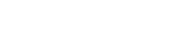This is the formula we are going to get at the end of this post. Although it may look crazy and you may be wondering how the heck can dy and dx be under the square root, the formula is actually very easy to understand: dy/dx means “derivative of y, i.e. of the function f(x), therefore you take the derivative and then you square it. Let’s say the function is f(x)=x²: the derivative is 2x, and once you square it you get 4x²; then you can proceed and solve the integral regurarly.

Now, imagine you approximate the area under a parabola with rectangles, like this:

What’s left is small red regions that look a lot like right triangles. You can see it better here:

The width of each triangle is called dx, since it is an infinitely small portion. It can also be called Δx, which indicates that it’s a “variation” of x. But since we are talking about integrals, that delta x is extremely small, so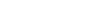The same is for dy, which is the height: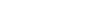ΔL is the hypotenuse of the right triangle and, again, it is so small that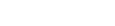We know, from the pythagorean theorem, that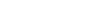In our case a is dx, b is dy and c is dL, therefore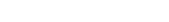Since we want to find L, we can take the integral on both sides (the integral of dL, i.e. of 1 with respect to L, is L):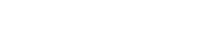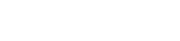You can understand this also by thinking that L is given by the sum of all dL‘s, hence the integral. One ΔL doesn’t approximate the curve very well, but many of it do, and to have many of them they need to be extremely small, therefore dL.

We know that we can’t integrate with respect to nothing; also, how can we know what dx and dy are? We can’t! BUT, when you need something, you can simply multiply by it, without forgetting to divide by it as well so that the expression stays the same. We need the dx, right? This means we will multiply everything by dx/dx: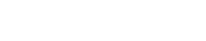We want just one dx in front of the function, therefore we can move the dx that is at the bottom inside the square root, but first we have to square it.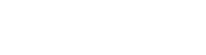Nothing has changed, we are just manipulating things in order to get what we want. At this point we can divide (dx)² and (dy)² by (dx)²: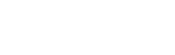or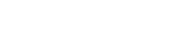We are almost there!

This gives you the formula to find the arc length, but if you have an interval, this can be written asor, in a more familiar and compact way, using the definite integraland this is the formula!

I really hope you liked this post and that everything was clear! If you have any questions, doubts, suggestions, leave a comment and I will be happy to reply!

Join 31 other subscribers.